• Topic Specification## Series and Parallel Circuits Revision

Try it yourself.

• Example Questions
• Worksheets 1## Filter by Level

Filter by tier, filter by exam board, series and parallel circuits.

Components can either be connected in series or parallel . The type of circuit can have an effect on the current , potential difference and resistance of the circuit.

## Series Circuits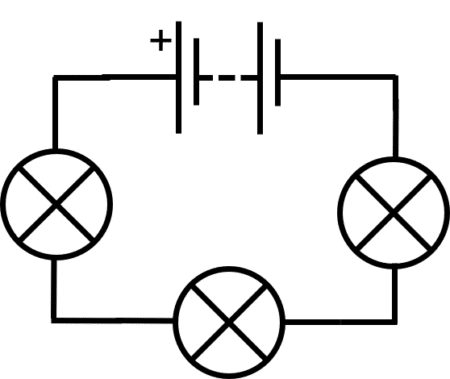In a series circuit , circuit components are connected in a single loop , one after another.

The same flow of charge passes through each circuit component and so the current is the same in every component:

\textcolor{aa57ff}{\text{I}_{\text{total}}=\text{I}_1=\text{I}_2=\text{I}_3=\text{...}}

The battery or cell provides the supply of potential difference to the circuit.  The potential difference is shared between the other components. So the total potential difference is equal to the potential difference of the battery:

\textcolor{aa57ff}{\text{V}_{\text{s}}=\text{V}_1+\text{V}_2+\text{V}_3+\text{...}}

Resistances in series adds up :

\textcolor{aa57ff}{\text{R}_{\text{total}}=\text{R}_1+\text{R}_2+\text{R}_3+\text{...}}

## Parallel Circuits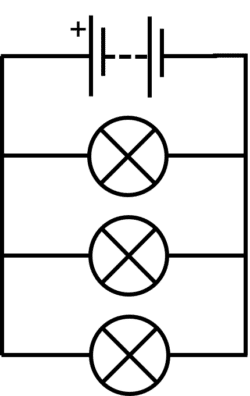In parallel circuits , components are connected in separate loops .

Each charge can only flow through one of the loops and so current is shared between loops . So the supply current in the loop with battery is equal to the sum of the current in the other loops:

\textcolor{f21cc2}{\text{I}_s=\text{I}_1+\text{I}_2+\text{I}_3+\text{...}}

The potential difference is the same in every loop :

\textcolor{f21cc2}{\text{V}_1=\text{V}_2=\text{V}_3}

When resistors are connected in parallel, the supply current is shared between the resistors and so the overall resistance is reduced as the electrical charges may take many paths.

## Example: Series and Parallel Circuit Rules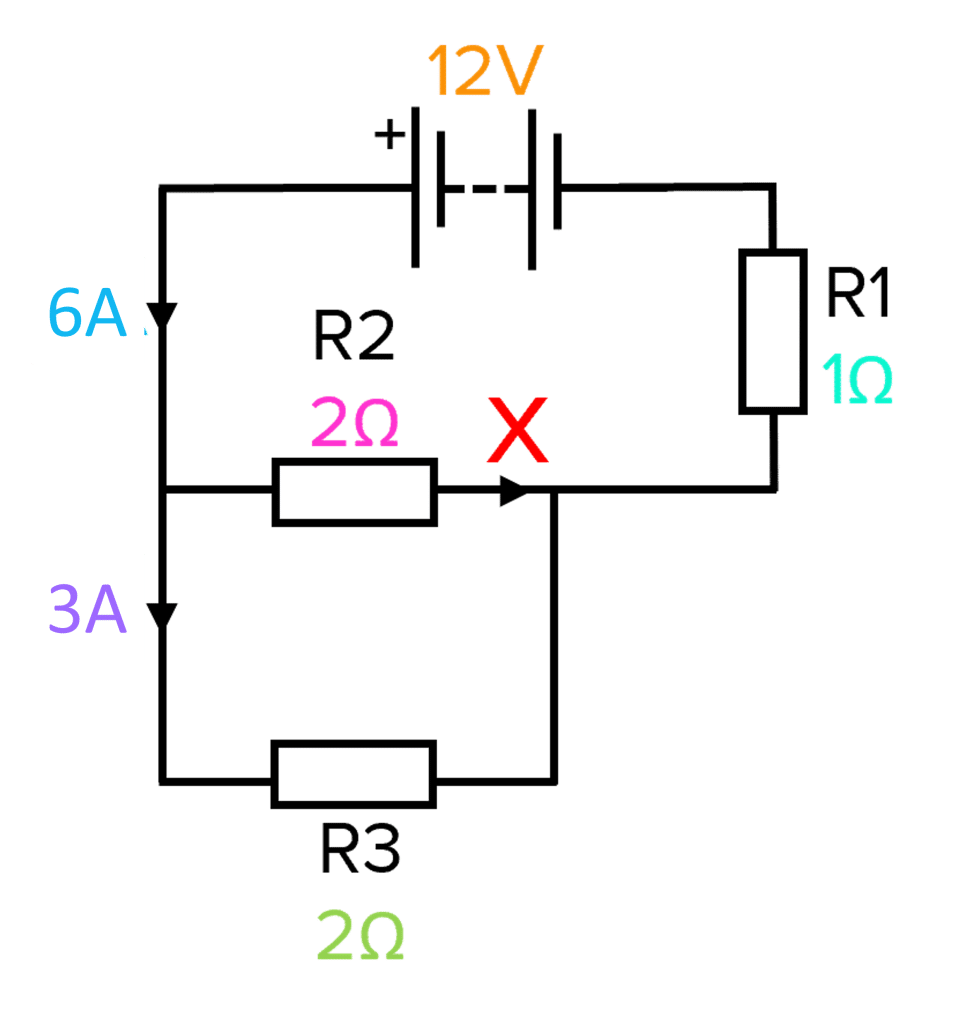A student builds a circuit containing a battery and three resistors named \text{R}1 , \text{R}2 and \text{R}3 . A diagram showing their circuit is shown on the right.

a)  Calculate the current at the arrow marked X on the circuit diagram.

b)  What is the potential difference across resistor \text{R}1 ?

c)  Suggest possible values for the potential difference across resistors \text{R}2 and \text{R}3 . Explain your answer.

a) In parallel, current is shared between loops so:

\bold{\text{I}_{\text{s}}=\text{I}_1+\text{I}_2} .

Rearrange for I 2 :

\text{I}_2=\text{I}_{\text{s}}-\text{I}_1=\textcolor{10a6f3}{6\text{ A}}-\textcolor{aa57ff}{3\text{ A}}=\bold{3\text{ A}}

b)   \bold{\text{R}=\dfrac{\text{V}}{\text{I}}}

Rearrange to find V:

\text{V}=\text{IR}=\textcolor{10a6f3}{6\text{ A}}\times \textcolor{00bfa8}{1 \, \Omega}=\bold{6\text{ V}}

c) R2 and R3 have the same resistance so potential difference must be equal .

\text{V}_1 = \text{V}_2=\text{V}_3=6\text{ V}

## Series and Parallel Circuits Example Questions

Question 1: State the difference between a series and parallel circuit.

In a series circuit, circuit components are connected in a single loop whereas in a parallel circuit, components are connected in multiple, separate loops .Gold Standard Education

Question 2: Two lamps are connected in series. The current in one lamp is 5\text{ A} . What is the current in the other lamp.

\bold{5\text{ A}}

In series, all components receive the same current.

Question 3: Two 3  \Omega resistors are connected in series. What is the total resistance of the circuit?

Question 4: A student connects three resistors in a series circuit, and then in a parallel circuit. Which circuit has the least overall resistance? Explain your answer.

The parallel circuit has the least overall resistance . This is because in series, each charge carrier must flow through each resistor and so the resistances add up . In parallel, the charge carriers have multiple possible paths and so resistance is less.

Question 5: Three lamps are connected in parallel to a battery that provides a current of 20\text{ A} . The current across two of the lamps is measured to be 5\text{ A} and 7\text{ A} . What current is measured across the third lamp?

\bold{\text{I}_{\text{s}}=\text{I}_1+\text{I}_2+\text{I}_3} (current is shared between the lamps)

Rearrange for I 3 :

## Series and Parallel Circuits Worksheet and Example Questions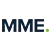## Series and Parallel Circuits Questions

£19.99 /month. Cancel anytime

## Where next?

Previous gcse physics topic, gcse physics revision home, go back to the main gcse physics topic list, next gcse physics topic, mains electricity.

By clicking continue and using our website you are consenting to our use of cookies in accordance with our Cookie Policy

## Report a Question

You must be logged in to vote for this question..

• International
• Schools directory
• Resources Jobs Schools directory News Search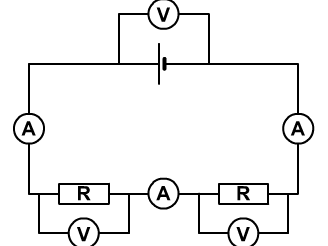## GCSE Physics - Parallel circuits: current and voltage - Circuits lesson 5

Subject: Physics

Age range: 14-16

Resource type: Worksheet/ActivityLast updated

24 June 2022

• Share through email
• Share through pinterestLesson describing the rules for current and voltage in a parallel circuit.

Includes easy to follow powerpoint, worksheet activities and answers written to make it easy for students to follow and self assess.

Creative Commons "Sharealike"

## Get this resource as part of a bundle and save up to 52%

A bundle is a package of resources grouped together to teach a particular topic, or a series of lessons, in one place.## GCSE Physics - Circuits - Complete lessons

Complete set of lessons (13 in total). At less than £1.50 a lesson, how much is your time worth? Each lesson has an easy to follow powerpoint, pupil activities, worksheets and answers. All ready to use. Also includes instructions for required practicals.

It's good to leave some feedback.

Something went wrong, please try again later.

## ZainabHashmi

Excellent resources!

Empty reply does not make any sense for the end user

Report this resource to let us know if it violates our terms and conditions. Our customer service team will review your report and will be in touch.

## Not quite what you were looking for? Search by keyword to find the right resource:## GCSE Physics OnlineHome    |    Premium Plan   |    Students   |    Parents    |    Teachers   |   Past Papers   |    A Level Physics   |    Shop

Physics Worksheets

## Worksheets to support all areas of GCSE Physics!    These are perfect for students to work through in lessons or independently at home. Each worksheet can be printed out for use in class or viewed on mobile devices - they also include a set of answers and a video with explanations and how to approach that style of question.Stores and Transfers

Efficiency

Kinetic Energy

Gravitational Potential Energy

Elastic Potential Energy

Power

Heat Transfer

Energy Resources

Drawing Circuits

Current and Charge

V, I and R

IV Characteristics

LDRs and Thermistors

Series Circuits

Parallel Circuits

Series and Parallel Circuits

Electrical Power

Energy Transfer in Circuits

Static Electricity

Density

Specific Heat Capacity 1

Specific Heat Capacity 2

Specific Latent Heat

Atoms and Isotopes

Nuclear Decay Equations

Half-life

Fission and Fusion

​      Identifying Forces

Free Body Diagrams

Resolving Forces

Work Done

Springs

Moments

Pressure at a Surface

Pressure in Fluids

Speed

Velocity

Acceleration

Distance-time Graphs

Velocity-time Graphs

Equations of Motion

Newton's Second Law

Stopping Distances

Momentum

Conservation of Momentum

Wave Speed, Period and Frequency

Reflection

Ultrasound Scanning

The EM Spectrum

Refraction

Convex (Converging) Lenses

Concave (Diverging) Lenses

Colour

Magnetic Fields

The Motor Effect

Generator Effect

Transformers 1

Transformers 2

Lifecycle of Stars

The Big Bang

## Electricity

These worksheets are provided for private study only and are not to be used for any financial gain.

## Circuits

Potential dividers, emf and internal resistance, resistivity, capacitors mc, capacitor energy, charge and discharge, uniform electric fields, electric fields, dr andrew gibson bsc pgce msc phd qts mr lander bennewith msc pgce qts.

Our availability rates and contact details.

Find out more about our background in physics and education.

After A-level and GSCE resources? Check out our free worksheets.

Take a look at how online tutoring works and other FAQs.

## List of GCSE worksheets## Topic 10 – Electricity and Circuits## University College London - MSc Pharmacology

Friendly and enthusiastic Science Tutor with 10+ years experience.Notes || Flashcards || Questions by Topic

• Definitions
• Summary Notes
• Current and Circuit Components
• Series and Parallel Circuits
• Mains Electricity

## Questions by Topic

2018-2021 papers.

• 10.1 Current and Circuit Components, Series and Parallel Circuits, and Resistance
• 10.2 Energy and Mains Electricity

## More Questions by Topic

Pre-2018 papers.

• Current, Voltage, Resistance, Energy & Power 1 MS
• Current, Voltage, Resistance, Energy & Power 1 QP
• Current, Voltage, Resistance, Energy & Power 2 MS
• Current, Voltage, Resistance, Energy & Power 2 QP
• Current, Voltage, Resistance, Energy & Power 3 MS
• Current, Voltage, Resistance, Energy & Power 3 QP
• Current, Voltage, Resistance, Energy & Power 4 MS
• Current, Voltage, Resistance, Energy & Power 4 QP
• Practical Electricity 1 MS
• Practical Electricity 1 QP
• Practical Electricity 2 MS
• Practical Electricity 2 QP
• Practical Electricity 3 MS
• Practical Electricity 3 QP
• Revision Courses
• Past Papers
• Solution Banks
• Numerical Reasoning
• Legal Notices#### IMAGES

1. AQA GCSE Physics (4.2.2) Electricity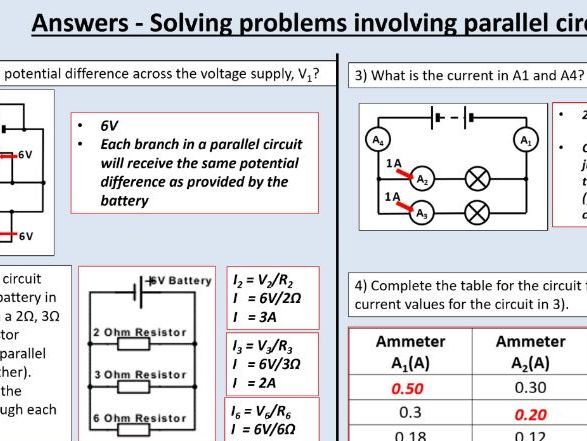2. Series Circuits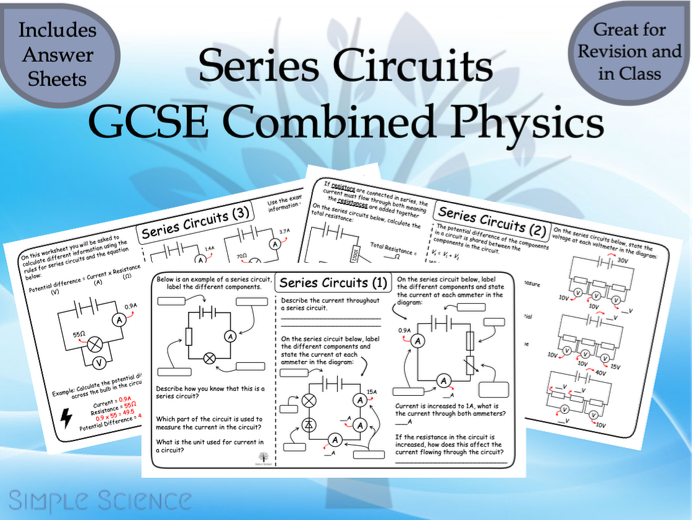3. Pin on Circuits science4. GCSE Physics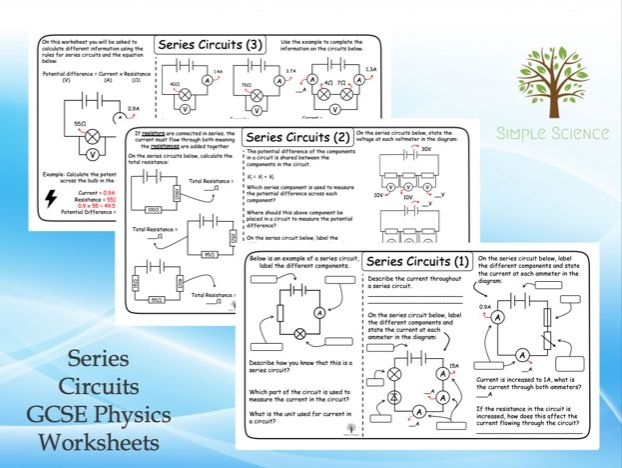5. GCSE 9-1 Physics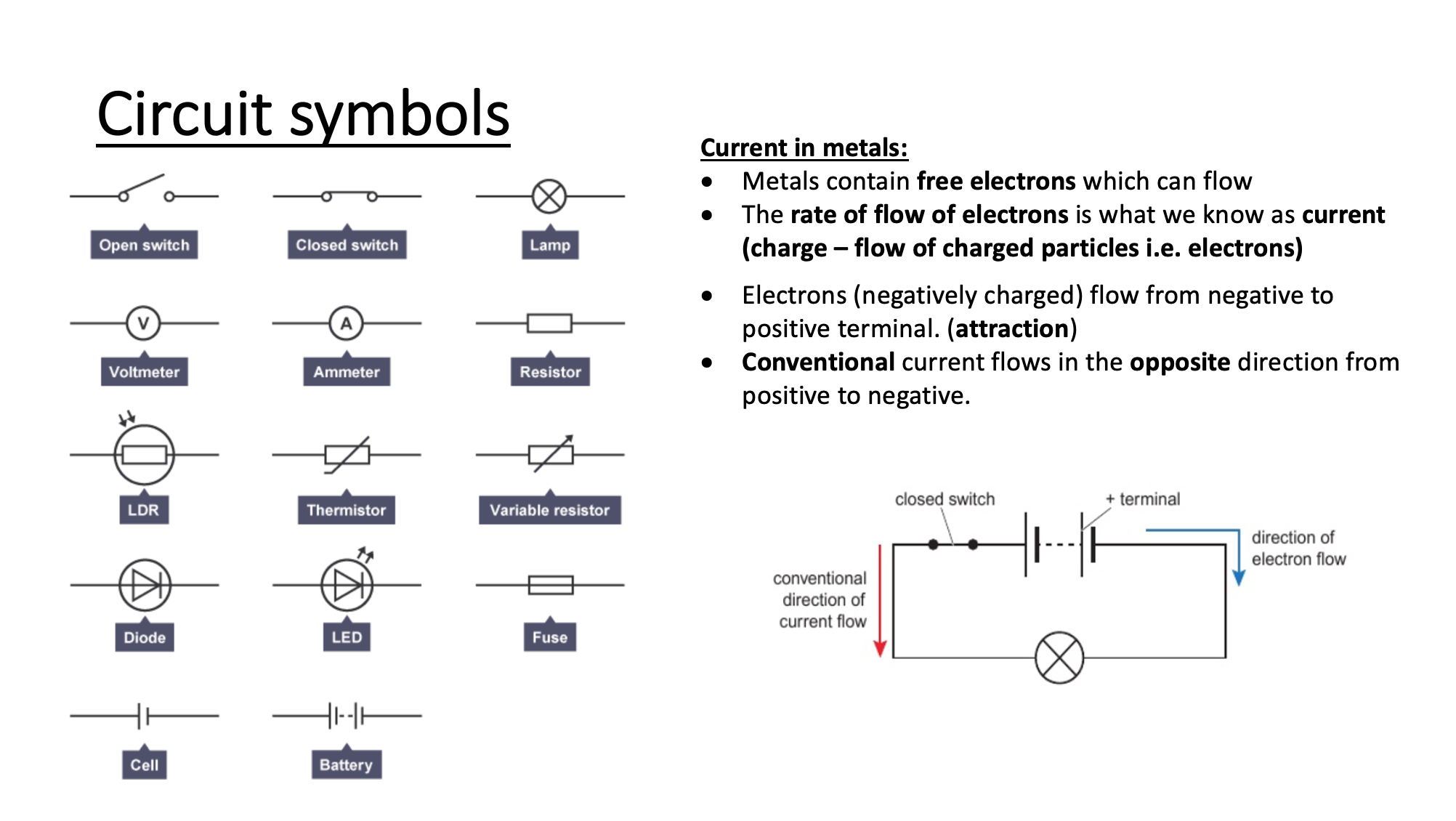6. Hand-drawn Science GCSE Circuits Summary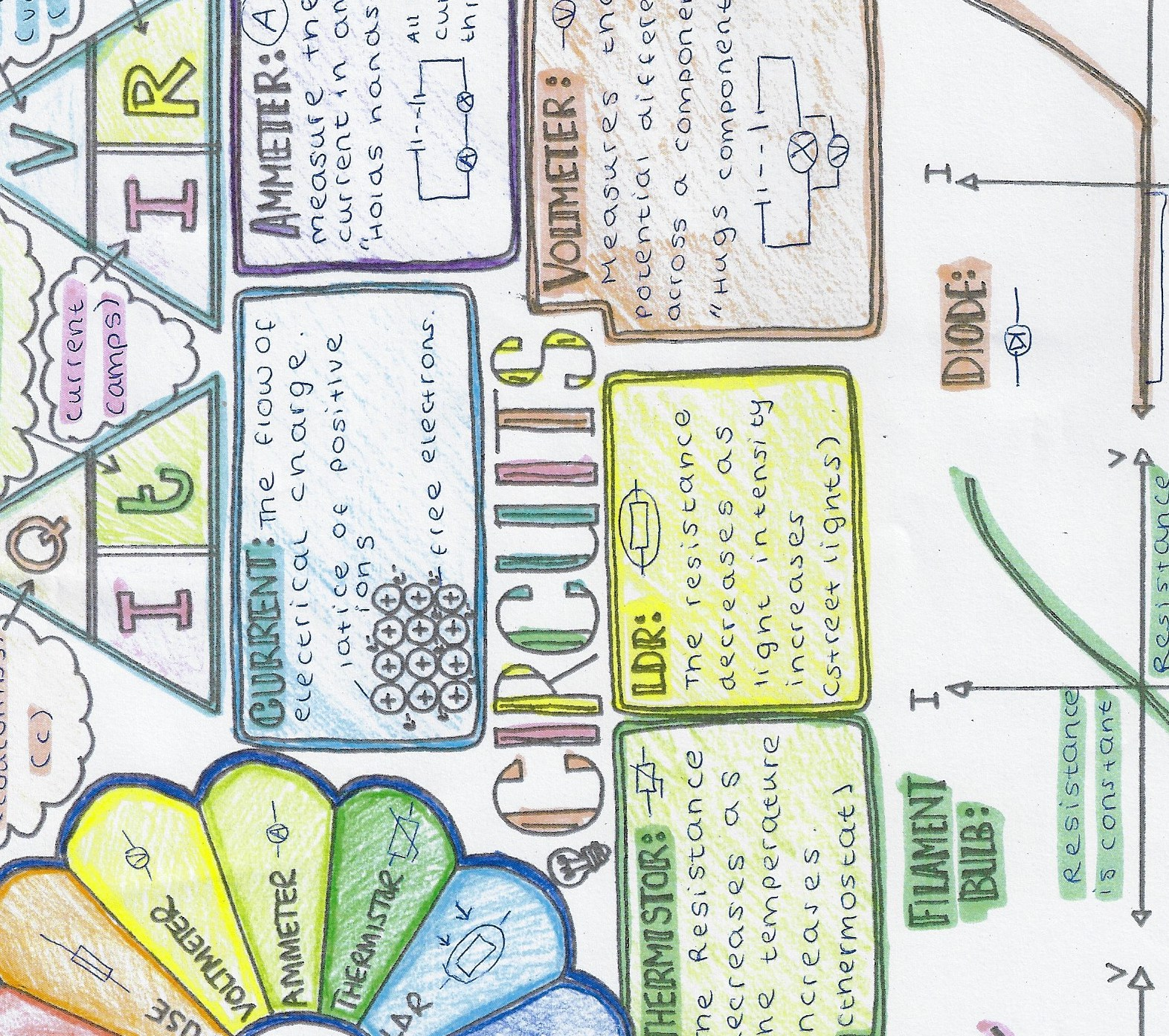#### VIDEO

1. 10 : CURRENT ELECTRICITY

2. 3rd Quarter MELC-Based Video Lesson in Science 5 (Open and Closed Circuit)

3. GCSE Physics: Electricity & Circuits

4. Circuits with more components

5. 07 potentiometer according to IGCSE

6. Ch3L3A Electric Current and Simple Circuits IGCSE GCSE Physics

1. Tips and Tricks for Making the Most of Teachers for Teachers Worksheets

Teachers for Teachers worksheets are a great way to provide students with engaging and educational activities. With the right approach, these worksheets can be used to help students learn and retain information in an effective way. Here are...

2. Make Math Fun with Engaging Math Practice Worksheets

Math can be a challenging subject for many students, but it doesn’t have to be. With the help of engaging math practice worksheets, you can make math fun and help your students develop their math skills. Here are some tips on how to make ma...

3. Where to Find Printable Reading Worksheets

Teaching children to read is an important skill they’ll use for the rest of their lives. When children need extra practice using their reading skills, it helps to have worksheets available. You can find an assortment of printable reading wo...

4. circuits

. TOTAL /12. Page 5. GCSE PHYSICS. High Demand Questions. CIRCUITS. QUESTIONSHEET 5. In the circuit below, 0.6 A flows through the 10 ohm resistor. 10 Ω. 20

5. Series and Parallel Circuits Worksheets, Questions and Revision

Series and parallel circuits worksheets, questions and revision for GCSE Combined Science and Physics. All the revision you need in one spot.

6. Series and Parallel Circuits

This worksheet is designed for GCSE Physics students. It includes a series of questions of increasing challenge, with answers and extra

7. GCSE Physics: Electricity

Resistance. How difficult it is for electrical charge to flow through a component. Measured in. Ohms. Power. The amount of energy transferred by a circuit each

8. GCSE Physics

Lesson describing the rules for current and voltage in a parallel circuit. Includes easy to follow powerpoint, worksheet activities and answers written to

9. Worksheets

Drawing Circuits · Current and Charge · V, I

10. GCSE Physics: Electricity and Circuits

The circuit below shows a lamp in series with an ammeter. A student wants to

11. AQA GCSE Physics Worksheets

AQA GCSE challenging worksheet for grade 9, high demand quesitons sorted by section.

12. Electricity worksheets, DC Circuits questions

Electricity problems and worksheet from past exam papers. This is the most challengin topic in AS physics.

13. List of GCSE worksheets

1 Current in series and parallel circuits (﻿Google Form version available

14. Edexcel GCSE Physics Topic 10: Electricity and Circuits Revision

Summary notes, revision videos and past exam questions by topic for Edexcel GCSE Physics Topic 10 - Electricity and circuits.